• SLIC超像素分割MATLAB代码SLIC 超像素 该存储库提供了带有 Python 和 Matlab 接口的简单线性迭代聚类 (SLIC) 算法的代码。 在这两种情况下，都提供了一个演示文件，应该很容易使用。 这两个版本都可以为灰色、彩色...
• 切片代码matlab C++ 代码来自 Chuan Yang、Lihe Zhang、Huchuan Lu、Xiang Ruan 和 Ming-Hsuan Yang 的论文“Saliency Detection via Graph-Based Manifold Ranking”。 发表于 IEEE 计算机视觉和模式识别会议 (CVPR...
• SLICmatlab代码，可以更改自己的图片位置，生成结果，可以直接运行使用
• 目前只看到SLIC的简介和C++代码，有兄弟评论需要matlab版，这里给大家找来了，记得给好评喔
• SLIC超像素分割算法MATLAB算法代码实现，超像素分割关键代码形式
• SLIC： simple linear iterative clustering的简称，即简单的线性迭代聚类。 这是一个基于聚类算法的超像素分割，由LAB空间以及x、y像素坐标共5维空间来计算。不仅可以分割彩色图，也可以兼容分割灰度图，它还有一...
• 简单，可运行，代码只有几行，直接调用函数，图片都有。
• SLIC超像素分割原作者只提供C++代码matlab遍寻不到。而超像素分割作为许多应用的第一步，不应该在其上花费太多功夫。鉴于C翻译成matlab比较麻烦运行又慢，最好的方式是混合编程，将C++程序封装好供matlab直接调用...
• 代码网址：http://ivrl.epfl.ch/research/superpixels#SLICO PS：上面的链接失效了，我搞了个代码托管，附链接：...里面的“SLIC_mex.zip”应该是作者官网上下载的。 下载了SLIC超像素分割的MATL...
更新：评论区有同学分享了新的代码链接，贴在这里吧：

https://www.epfl.ch/labs/ivrl/research/slic-superpixels/

***************************************************   分       割        线    ******************************************************

代码网址：http://ivrl.epfl.ch/research/superpixels#SLICO

里面的“SLIC_mex.zip”应该是作者官网上下载的。

下载了SLIC超像素分割的MATLAB程序：

“Mex file and MATLAB demo script”

下载解压之后，主程序是“SLICdemo.m”，它会调用C语言程序“slicmex.c”。

所以运行程序的时候，首先通过MATLAB将C/C++函数编译成MEX函数，在MATLAB中就可以调用了。方法是：

MATLAB的command window下输入：

>>mex silcmex.c

会通知你选择编译器：

Select a compiler:
 Microsoft Visual C++ 2010 in d:\ruanjiananzhuang\VS2010UltimTrialCHS

 None

Compiler: 1

输入1，回车，这时就会报出警告和错误，警告不用管，主要解决错误：

slicmex.c(387) : error C2275: “mwSize”: 将此类型用作表达式非法

......

这个错误是最先出现的最大的错误，先解决它。

用文本编辑器打开文件“slicmex.c”，处理（387）行：

mwSize numdims = mxGetNumberOfDimensions(prhs) ;

改法就是：将numdims的声明和定义拆开：

mwSize numdims;

numdims = mxGetNumberOfDimensions(prhs) ;

再将其声明与（351）行定义的mexFunction函数下面的一大串变量声明放在一起。截图如下：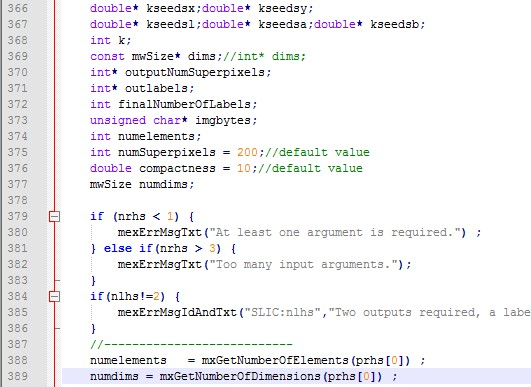保存文件，再返回到MATLAB的命令窗口处，重新编译c程序：

>> mex slicmex.c

再运行程序：
>> SLICdemo

网上有人给出了原理，我把文字复制过来：

地址：http://www.cppblog.com/colorful/archive/2015/02/05/209744.aspx

error C2275: “XXX”: 将此类型用作表达式非法

在移植c++代码到c的时候，经常会出现一个奇怪的错误，
error C2275: “XXX”: 将此类型用作表达式非法
表达式非法，这个错误是由于c的编译器要求将变量的申明放在一个函数块的头部，而c++没有这样的要求造成的。
解决的办法就是把变量的申明全部放在变量的生存块的开始。


展开全文• 图像模糊matlab代码Fuzzy SLIC (Precise superpixel number control version) with Matlab Interface的C实现 版权所有 (c) 2018, Chong WU 保留所有权利。 如果满足以下条件，则允许以源代码和二进制形式重新分发和...
• SLIC超像素分割MATLAB代码夜间天空/云图像分割 本着可重复研究的精神，该存储库包含在手稿中产生结果所需的所有代码：S. Dev、FM Savoy、YH Lee 和 S. Winkler，夜间天空/云图像分割， Proc。 IEEE 国际图像处理会议...
• 图像模糊matlab代码带有 Matlab 接口的模糊 SLIC 的 C 实现 汽车密实系数选择版 版权所有 (c) 2019, Chong WU 保留所有权利。 如果满足以下条件，则允许以源代码和二进制形式重新分发和使用，无论是否修改： 源代码...
• 1. 说明　关于超像素分割的背景不在过多说明，大致作用就是将区域内相似属性的超像素集合到一起，...2. 下载地址和使用说明　SLIC算法C++/Matlab的demo可以下面链接中下载： 　http://ivrl.epfl.ch/research/superpix
1. 说明

关于超像素分割的背景不再过多说明，大致作用就是将区域内相似属性的像素集合到一起，合成一个有更多属性的块区域。其中SLIC算法应用最广泛，现在在图像处理和计算机领域有很广泛的应用。作用如图所示：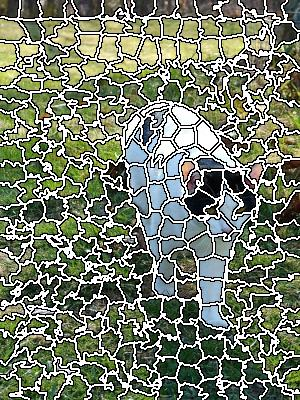2. 下载地址和使用说明

SLIC算法C++/Matlab的demo可以下面链接中下载：
http://ivrl.epfl.ch/research/superpixels 　　至于matlab下使用说明也不在过多描述，直接摘自原程序中说明：

%Input parameters are:
% 8 bit images (color or grayscale)
% Number of required superpixels (optional, default is 200)
% Compactness factor (optional, default is 10)
%
%Ouputs are:
% labels (in raster scan order)
% number of labels in the image (same as the number of returned
%superpixels
%
%NOTES:
% number of returned superpixels may be different from the input
%number of superpixels.
% you must compile the C file using mex slicmex.c before using the code
%below
%======================================================================
[labels, numlabels] = slicmex(img,200,20);%numlabels is the same as number of superpixels
imshow(labels);

3.Matlab程序的Bug修改

但是源程序slicmex.c在windows系统下编译是有错误的，主要提示如下：

slicmex.c(387) : error C2275: “mwSize”:将此类型用作表达式非法.

这是一个很典型的纯c程序的错误，尤其在移植c++代码到c的时候，经常会出现一个奇怪的错误，error C2275: “XXX”: 将此类型用作表达式非法。 　　表达式非法，这个错误是由于c的编译器要求将变量的申明放在一个函数块的头部，而c++没有这样的要求造成的。 　　修改如下： 　　将源代码中387行的 mwSize定义的类型变量放到该函数的开头，和函数中其他变量定义在一起。如图所示：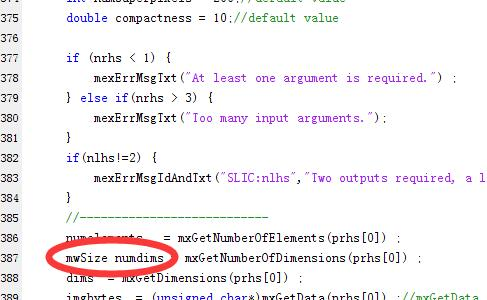修改成：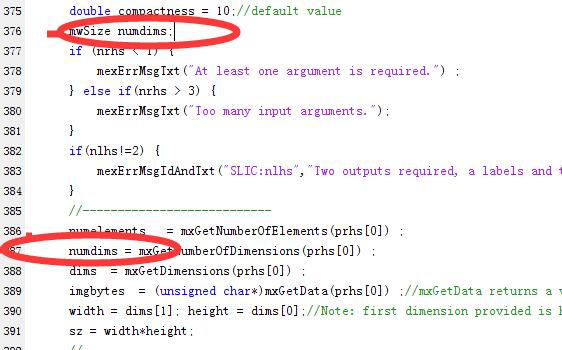如果还不知道怎么修改的话，请点击下面的连接下载修改过的slicmex.c文件：
https://github.com/DUTFangXiang/SLIC__superpixelSegmentation　　 　　同样，另外一个slicomex.c也是同样的一个问题，主要是变量没有定义在mexFunction函数的开头，它将判断输入是否正确写在了最前面，问题都集中在391行后面30行内，你可以自己将定义变量的地方移到函数的开头中，像文章中一样。 　　下载完成直接 mex slicmex.c即可，至于怎么配置mex环境我就不再过多叙述了，因为那又是一个漫长的话题。

个人学习记录，由于能力和时间有限，如果有错误望读者纠正，谢谢！


展开全文超像素分割
• 首先介绍vlfeat库函数：vl_slic,vl_quickshift，vl_quckseg vl_slic SLIC superpixels segments = vl_slic(im,regionsize,regularizer) segments：每个像素值是超像素标号 regionsize：超像素的起始大小 ...
首先介绍vlfeat库函数：vl_slic,vl_quickshift，vl_quckseg

vl_slic  SLIC superpixels

segments = vl_slic(im,regionsize,regularizer)
segments：每个像素值是超像素标号
regionsize：超像素的起始大小
regularizer：用于聚类时控制超像素呈现的空间规则性，值越大超像素块越规则
im：需要转为single类型
vl_quickshift  Quick shift image segmentation

[map,gaps] = vl_quickshift(im, kernelsize, maxdist)
[map, gaps] = vl_quickshift(I, kernelsize)
[map, gaps,e] = vl_quickshift(I, kernelsize, maxdist)
kernelsize：估计density的Parzen window的bandwidth
maxdist：控制所连接邻域的最大L2距离
map和gaps代表结果forest的trees：map每个像素表示forest的parent元素，gaps包含对应的分支长度
vl_quickseg  Produce a quickshift segmentation of a grayscale or color image

vl_quickseg要调用vl_quickshift
[iseg, labels, maps, gaps, e] = vl_quickseg (im, ratio, kernelsize, maxdist)
iseg：color image。每个像素被其区域平均color标记
labels：labeled image。标号为类别号
maps：pointer。指向最近的增大其density估计值的像素
gaps：distance。距最近的增大其density估计值的像素的距离
e：density的估计值
im：需要转换为double类型
kernelsize：标准差
maxdist：tree nodes之间的最大距离。用于cut links in the tree to 构成分割结果
ratio：空间一致性和颜色一致性之间的比率。ratio越小空间比重越大，空间距离在归一化之前计算。类似vl_slic参数regularizer，不过ratio越小超像素块越规则
对于vl_slic得到的segments或者labels，可以有以下两种方式显示超像素分割结果

1、以颜色标记形式显示：imagesc();

imagesc(SEGMENTS);  %Scale data and display image object
axis equal off tight; %关闭坐标
colormap gray;  %以灰度图形式显示

[sx,sy]=vl_grad(double(SEGMENTS), 'type', 'forward') ; %求梯度
s = find(sx | sy) ;  % or - Find logical OR
imp = im ;
imp([s s+numel(im(:,:,1)) s+2*numel(im(:,:,1))]) = 255 ;  %边界置为白色
imshow(imp);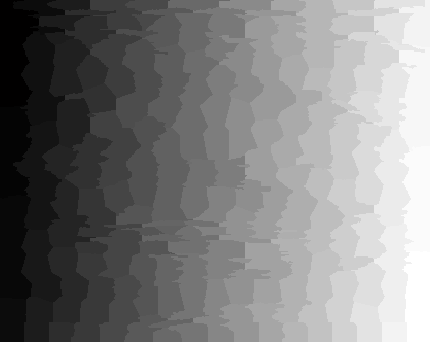颜色形式。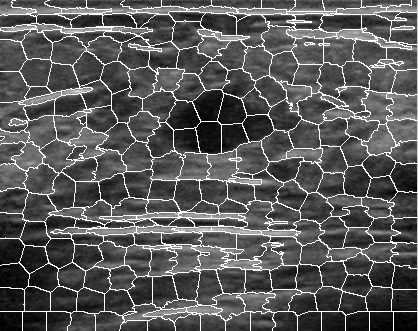边界形式。

%完整代码
REGIONSIZE=25;
REGULARIZER=0.05;
[m,n,c]=size(im);
if(c==1)
im=cat(3,im,im,im);
end
I =  vl_rgb2xyz(im);
I_single = single(I);
SEGMENTS = vl_slic(I_single, REGIONSIZE, REGULARIZER);
imagesc(SEGMENTS);
axis equal off tight;
colormap gray;
s = find(sx | sy) ;
imp = im ;
imp([s s+numel(im(:,:,1)) s+2*numel(im(:,:,1))]) = 255 ;
figure;imshow(imp);


展开全文• matlab灰度处理代码 ###################################################### #######方法1：superpixels_seeds##### ###################################################### 超像素分割方法，采用论文 SEEDS: ...
• matlab超像素代码，直接运行main函数可运行，k表示超像素块的个数
• SLIC超像素分割MATLAB代码演示_SSAL_SDP 所提出的 SSAL-SDP 方法的演示，即基于超像素的半监督主动学习 (SSAL) 方法与密度峰值 (DP) 增强，用于高光谱图像分类。 这组文件包含用于基于超像素的半监督主动学习 (SSAL)...
• 代码为C代码、需编译成MATLAB可执行文件后使用。经本人使用验证有效。。有问题的可以私信。。该代码确定可以实现相应的功能。。
• matlab代码间距关于 Matlab Mex文件，用于生成3D SLIC超体素。 从C ++实现改编的Supervoxel代码。 预期输入 3D灰度图像uint32。 附加参数 添加了间距参数，以允许在x，y和z方向上具有不同的间距。 有关在3D图像上...
• 代码中使用的算法是对 Achanta 等人提出的简单线性迭代聚类 (SLIC) 方法的修改。 (2012)。 我们的方法针对 MRI、CT 等医学图像进行了优化。我们的代码与传统 2D 和 3D 超像素相比的贡献如下： • 多模式输入（也...
• ## 超像素分割算法SLIC的matlab实现

千次阅读 多人点赞 2020-08-20 16:59:05
SLIC是一种基于网格化KMeans聚类的超像素分割算法，其计算复杂度为O(N)，其中N为像素点个数。SLIC的原理比较简单精致，具体的原理我这里就不介绍了，推荐大家自己去读原始论文加深理解。SLIC的算法流程如下： 如...
SLIC是一种基于网格化KMeans聚类的超像素分割算法，其计算复杂度为O(N)，其中N为像素点个数。SLIC的原理比较简单精致，具体的原理我这里就不介绍了，推荐大家自己去读原始论文加深理解（但我以为看下面这个算法流程图就足够理解原理了）。SLIC的算法流程如下：如所有其他聚类算法一样，SLIC不能保证连通性，所以需要后处理将旁生的连通域合并到邻近的主连通域上，但是论文中并未给出具体的后处理方法。我给出的方法是按照轮廓接触点个数最多原则合并连通域。由于每个聚类都有自己的“势力范围”，即每个标签覆盖的区域不会超过聚类时限定的范围（一般是2S*2S大小，边缘栅格的聚类例外），所以合并处理时只需要在该范围内操作即可。

下面给出本人实现的SLIC算法程序（控制色域与空域权重比例的系数wDs应设为函数形参，这里就不改了。注意！迭代中限定聚类的栅格是不变的！！！）：

function Label=SLIC(img,s,errTh,wDs)
% 基于KMeans的超像素分割
% img为输入图像，维度不限，最大值为255
% s x s为超像素尺寸
% errTh为控制迭代结束的联合向量残差上限
m=size(img,1);
n=size(img,2);

%% 计算栅格顶点与中心的坐标
h=floor(m/s);
w=floor(n/s);
rowR=floor((m-h*s)/2); %多余部分首尾均分
colR=floor((n-w*s)/2);
rowStart=(rowR+1):s:(m-s+1);
rowStart(1)=1;
rowEnd=rowStart+s;
rowEnd(1)=rowR+s;
rowEnd(end)=m;
colStart=(colR+1):s:(n-s+1);
colStart(1)=1;
colEnd=colStart+s;
colEnd(1)=colR+s;
colEnd(end)=n;
rowC=floor((rowStart+rowEnd-1)/2);
colC=floor((colStart+colEnd-1)/2);
% 显示划分结果
temp=zeros(m,n);
temp(rowStart,:)=1;
temp(:,colStart)=1;
for i=1:h
for j=1:w
temp(rowC(i),colC(j))=1;
end
end
figure,imshow(temp);
imwrite(temp,'栅格.bmp');

%% 计算梯度图像，使用sobel算子和欧式距离
img=double(img)/255;
r=img(:,:,1);
g=img(:,:,2);
b=img(:,:,3);
Y=0.299 * r + 0.587 * g + 0.114 * b;

f1=fspecial('sobel');
f2=f1';
gx=imfilter(Y,f1);
gy=imfilter(Y,f2);
G=sqrt(gx.^2+gy.^2);

%% 选择栅格中心点3*3邻域中梯度最小点作为起始点
rowC_std=repmat(rowC',[1,w]);
colC_std=repmat(colC,[h,1]);
rowC=rowC_std;
colC=colC_std;
for i=1:h
for j=1:w
block=G(rowC(i,j)-1:rowC(i,j)+1,colC(i,j)-1:colC(i,j)+1);
[minVal,idxArr]=min(block(:));
jOffset=floor((idxArr(1)+2)/3);
iOffset=idxArr(1)-3*(jOffset-1);
rowC(i,j)=rowC(i,j)+iOffset;
colC(i,j)=colC(i,j)+jOffset;
end
end

%% KMeans超像素分割
Label=zeros(m,n)-1;
dis=Inf*ones(m,n);
M=reshape(img,m*n,size(img,3)); %像素值重排
% 联合色域值和空域值
colorC=zeros(h,w,size(img,3));
for i=1:h
for j=1:w
colorC(i,j,:)=img(rowC(i),colC(j),:);
end
end
uniMat=cat(3,colorC,rowC,colC);
uniMat=reshape(uniMat,h*w,size(img,3)+2);
iter=1;
while(1)
uniMat_old=uniMat;
%     rowC_old=rowC;
%     colC_old=colC;
for k=1:h*w
c=floor((k-1)/h)+1;
r=k-h*(c-1);
rowCidx=rowC(r,c);
colCidx=colC(r,c); %聚类中心坐标
%聚类限定的栅格(中心点始终是原s x s栅格的中心点)
rowStart=max(1,rowC_std(r,c)-s);
rowEnd=min(m,rowC_std(r,c)+s-1);
colStart=max(1,colC_std(r,c)-s);
colEnd=min(n,colC_std(r,c)+s);
%         colorC=uniMat(k,1:size(img,3));
colorC=M((colCidx-1)*m+rowCidx,:);
for i=rowStart:rowEnd
for j=colStart:colEnd
colorCur=M((j-1)*m+i,:);
dc=norm(colorC-colorCur);
ds=norm([i-rowCidx,j-colCidx]);
d=dc^2+wDs*(ds/s)^2;
if d<dis(i,j)
dis(i,j)=d;
Label(i,j)=k;
end
end
end
end

%显示聚类结果
temp=mod(Label,20)+1;
figure;
imagesc(label2rgb(temp-1,'jet','w','shuffle')) ;
axis image ; axis off ;
% 录制gif
F=getframe(gcf);
I=frame2im(F);
[I,map]=rgb2ind(I,256);
if iter == 1
imwrite(I,map,'test.gif','gif','Loopcount',inf,'DelayTime',0.2);
else
imwrite(I,map,'test.gif','gif','WriteMode','append','DelayTime',0.2);
end
iter=iter+1;

% 更新聚类中心
colorC=zeros(h,w,size(img,3));
for k=1:h*w
num=0;
sumColor=zeros(1,size(img,3));
sumR=0;
sumC=0;
c=floor((k-1)/h)+1;
r=k-h*(c-1);
rowCidx=rowC_std(r,c);
colCidx=colC_std(r,c);
rowStart=max(1,rowCidx-s);
rowEnd=min(m,rowCidx+s-1);
colStart=max(1,colCidx-s);
colEnd=min(n,colCidx+s);

for row=rowStart:rowEnd
for col=colStart:colEnd
if Label(row,col)==k
num=num+1;
sumR=sumR+row;
sumC=sumC+col;
color=reshape(img(row,col,:),1,size(img,3));
sumColor=sumColor+color;
end
end
end
colorC(r,c,:)=sumColor/num;
rowC(r,c)=round(sumR/num);
colC(r,c)=round(sumC/num);
end
uniMat=cat(3,colorC,rowC,colC);
uniMat=reshape(uniMat,h*w,size(img,3)+2);
diff=uniMat-uniMat_old;
diff(:,1:2)=sqrt(wDs)*diff(:,1:2)/s;
err=norm(diff)/sqrt(h*w);
if err<errTh %残差低于阈值，结束迭代
break;
end
end

%% 后处理， 按照边界接触点数最多原则分配小连通域的标签
for k=1:h*w
c=floor((k-1)/h)+1;
r=k-h*(c-1);
rowCidx=rowC_std(r,c);
colCidx=colC_std(r,c);
rowStart=max(1,rowCidx-s);
rowEnd=min(m,rowCidx+s-1);
colStart=max(1,colCidx-s);
colEnd=min(n,colCidx+s);
block=Label(rowStart:rowEnd,colStart:colEnd);
block(block~=k)=0;
block(block==k)=1;
label=bwlabel(block);
szlabel=max(label(:)); %标签个数
bh=rowEnd-rowStart+1;
bw=colEnd-colStart+1;  %block的宽高

if szlabel<2  %无伴生连通域，略过
continue;
end

labelC=label(rowCidx-rowStart+1,colCidx-colStart+1); %主连通域的标记值
top=max(1,rowStart-1);
bottom=min(m,rowEnd+1);
left=max(1,colStart-1);
right=min(n,colEnd+1);
for i=1:szlabel %遍历连通域
if i==labelC %主连通域不处理
continue;
end
marker=zeros(bottom-top+1,right-left+1); %生成一个外扩一圈的marker，标记哪些点已经被统计过接触情况
bw=label;
bw(bw~=i)=0;
bw(bw==i)=1; %当前连通域标记图
contourBW=bwperim(bw); %求取外轮廓
%             figure,imshow(contourBW);
idxArr=find(double(contourBW)==1);
labelArr=zeros(4*length(idxArr),1);  %记录轮廓点的4邻域点标记值的向量
num=0;
for idx=1:size(idxArr) %遍历轮廓点,统计其4邻域点的标记值
bc=floor((idxArr(idx)-1)/bh)+1;
br=idxArr(idx)-bh*(bc-1); %轮廓点在block中的行列信息
row=br+rowStart-1;
col=bc+colStart-1; %轮廓点在大图中的行列信息
rc=[row-1,col;...
row+1,col;...
row,col-1;...
row,col+1];
for p=1:4
row=rc(p,1);
col=rc(p,2);

if ~(row>=1 && row<=m && col>=1 && col<=n && Label(row,col)~=k)
continue;
end

if marker(row-top+1,col-left+1)==0 %未被统计过
marker(row-top+1,col-left+1)=1;
num=num+1;
labelArr(num)=Label(row,col);
end
end
end

labelArr(find(labelArr==0))=[]; %去除零元素
uniqueLabel=unique(labelArr);
numArr=zeros(length(uniqueLabel),1);
for p=1:length(uniqueLabel)
idx=find(labelArr==uniqueLabel(p));
numArr(p)=length(idx);
end
idx=find(numArr==max(numArr));
maxnumLabel=uniqueLabel(idx(1)); %接触最多的标签

for row=rowStart:rowEnd
for col=colStart:colEnd
if bw(row-rowStart+1,col-colStart+1)==0
continue;
end
Label(row,col)=maxnumLabel;
end
end
end
end

% 显示连通域处理后聚类结果
temp=mod(Label,20)+1;
figure;
imagesc(label2rgb(temp-1,'jet','w','shuffle')) ;
axis image ; axis off ;

脚本文件：

close all;clc;
figure,imshow(I);

s=15;
errTh=10^-2;
wDs=0.5^2;
Label=SLIC(I,s,errTh,wDs);

%% 显示轮廓
marker=zeros(size(Label));
[m,n]=size(Label);
for i=1:m
for j=1:n
top=Label(max(1,i-1),j);
bottom=Label(min(m,i+1),j);
left=Label(i,max(1,j-1));
right=Label(i,min(n,j+1));
if ~(top==bottom && bottom==left && left==right)
marker(i,j)=1;
end
end
end
figure,imshow(marker);

I2=I;
for i=1:m
for j=1:n
if marker(i,j)==1
I2(i,j,:)=0;
end
end
end
figure,imshow(I2);

测试图像：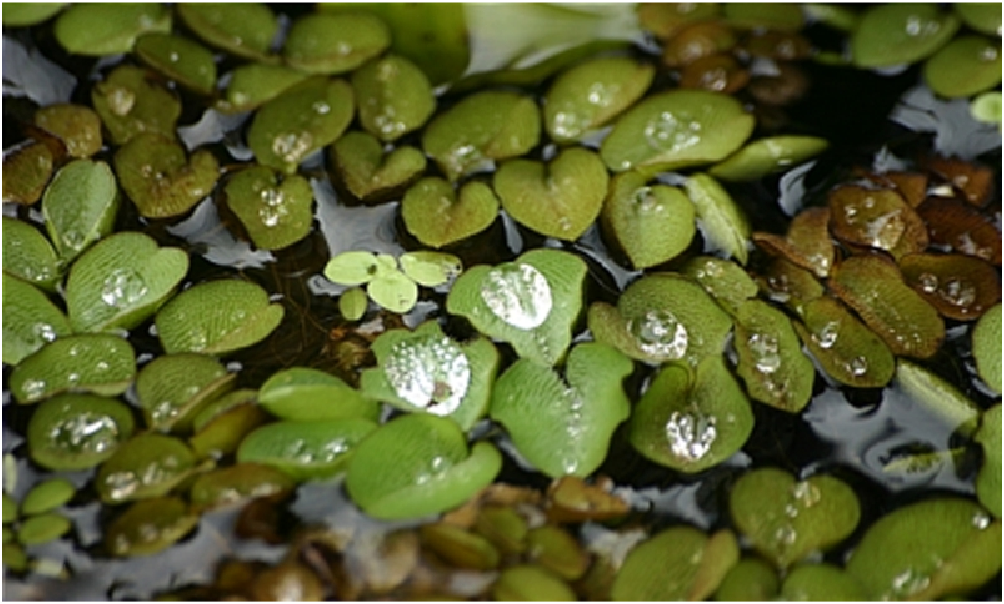栅格划分结果：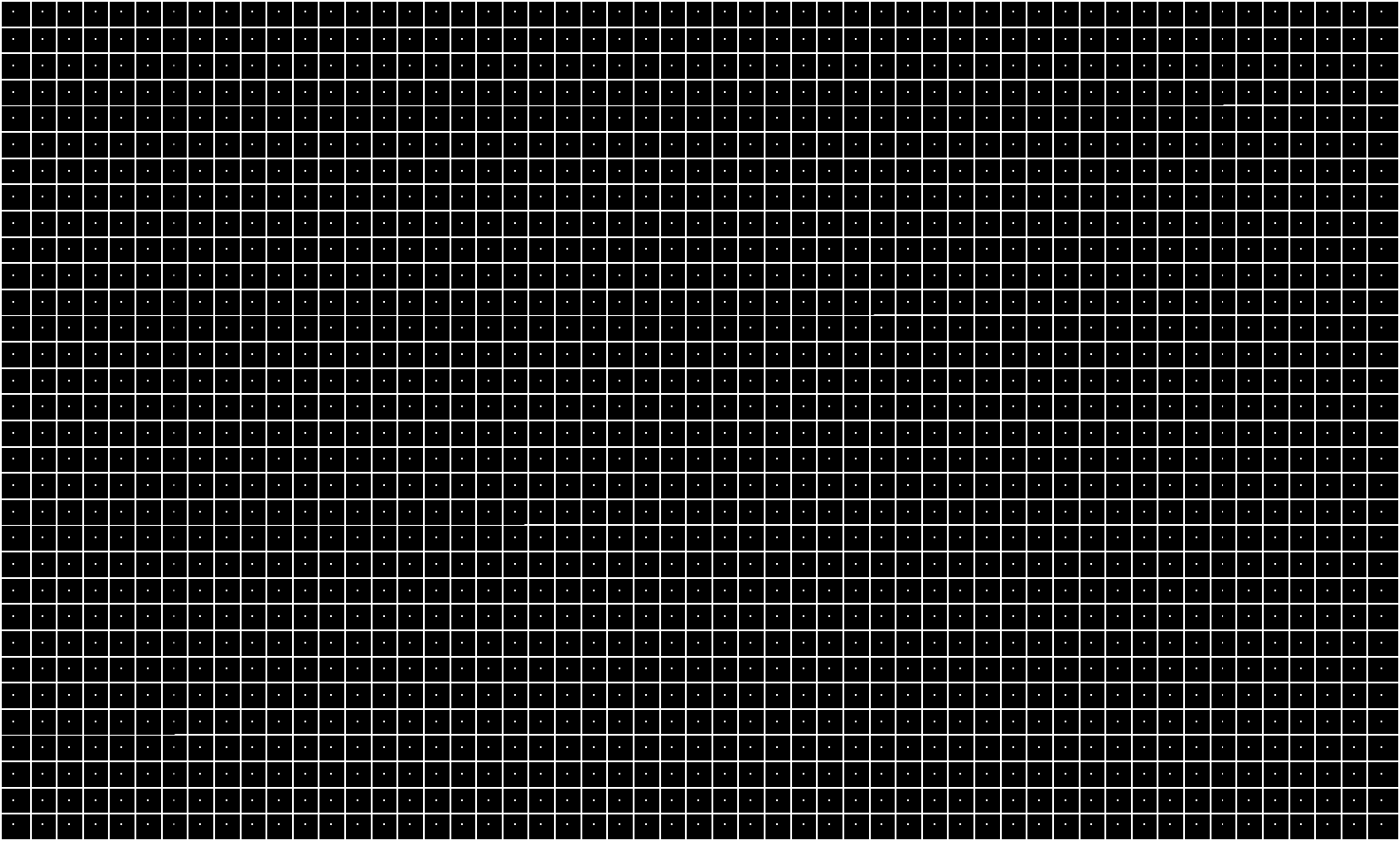聚类过程：聚类最终结果：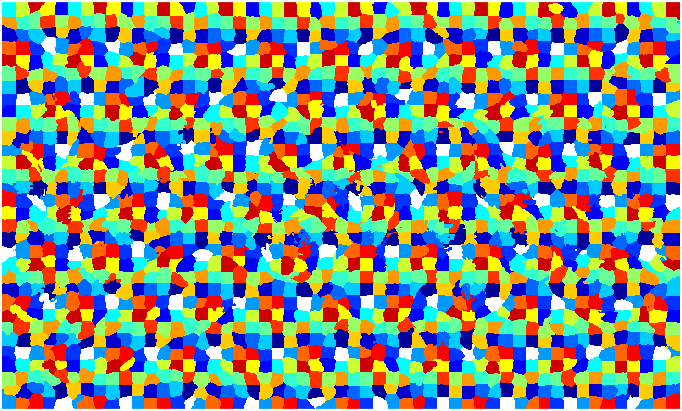连通域合并后的结果：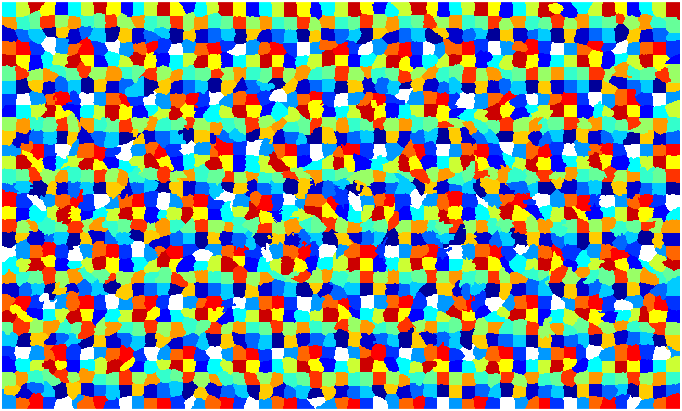原图+轮廓线：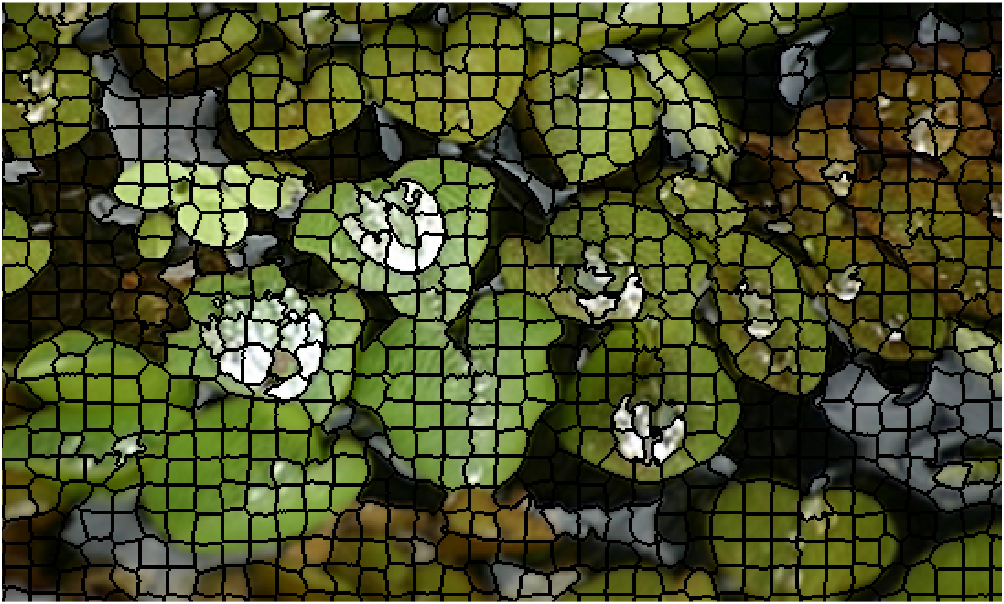具体的我就不解释了，自觉程序写得还是很有条理的，读者自己跟踪程序运行进行理解吧。


展开全文• 源码分析：作者上传的并不是纯粹的matlab代码，而是采用c写的生成的mex文件。 使用前提：我的电脑安装Matlab 2016ra和Vs2015 首先在下载源码的文件夹下面打开matlab，要写实现c和matlab混编，需要先配置，在命令行敲...
• 小白第一篇原创（算是原创吧哈哈哈哈），转载请注明出处附上原网址：...最初接触Matlab和C/C++的混合编程，是在用Matlab做图像处理的SLIC超像素分割，源码里面用到了Matlab和C混合编程。...混合编程
• 1、网上下载SLIC超像素分割的代码以及GUI超像素分割结果图的代码，或者直接到我网盘通过提取码下载 链接链接：https://pan.baidu.com/s/1I8r6o6Pang44iMTqKUzObQ 提取码：ywtr 2、打开matlab(我用的matlab R2016a)...
• C编译器用户必须自己在MATLAB中为SLIC超像素构建二进制MEX文件（请参见下文），这需要MATLAB支持的C编译器。 用于运行演示的安装 我们的雾仿真管道使用，对于，算法以a的形式实现，以便在MATLAB中使用。 简而言之，......

# matlabslic代码matlab 订阅